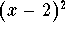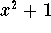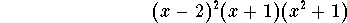#### Exercise 7.

Give an example of a polynomial of degree 5, whose only real roots are x=2 with multiplicity 2, and x=-1 with multiplicity 1.

We need factors of the formand (x+1) to satisfy the root requirements. We also know that the last 2 of the 5 roots of the polynomial have to be complex; so, for instance,will do:

The polynomialsatisfies all the requirements.

N.B.: In problems like this one, do not bother to multiply out. You were just asked to write down a polynomial with certain properties; nobody told you to write down the polynomial in a particular form.[Back] [Exercises] [Next]
[Algebra] [Trigonometry] [Complex Variables]
[Calculus] [Differential Equations] [Matrix Algebra]S.O.S MATHematics home page

Do you need more help? Please post your question on our S.O.S. Mathematics CyberBoard.Helmut Knaust
Tue Jun 24 09:53:41 MDT 1997